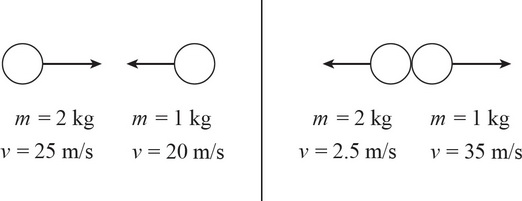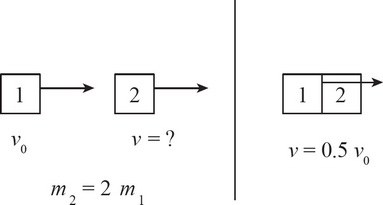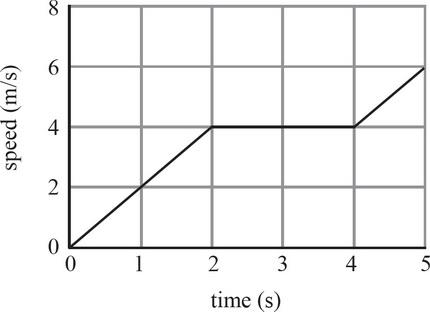# AP Physics 1 Multiple-Choice Practice Test 30

### Test Information10 questions18 minutes

1. A 2000 kg truck traveling north at 40.0 m/s collides with a 1500 kg car traveling west at 20.0 m/s. If the two vehicles lock bumpers and stick together, what is the angle of the velocity after impact?2. A 2 kg ball traveling at 25 m/s collides head on with a 1 kg ball traveling at 20 m/s. After impact, both objects reverse direction with the 2 kg ball traveling at 2.5 m/s and the 1 kg ball traveling at 35 m/s. What type of collision occurred?

3. Which of the following statements is FALSE regarding perfectly inelastic collisions?

4. A 0.145 kg baseball is travelling at 40 m/s horizontally when it is struck by a baseball bat. The baseball leaves the bat at 50 m/s back in the direction it came from, but at an angle of 40° above the horizontal. What is the magnitude of the impulse imparted to the baseball?5. Object 1 travels with an initial speed of v0 toward Object 2, which is traveling in the same direction as Object 1. Object 1 collides perfectly inelastically into Object 2 and the velocity after impact is 0.5v0. If Object 2 has twice the mass of Object 1, what must have been the initial speed of Object 2 (assuming no external forces)?

6. A 2 kg object is tied to a machine with a 0.5 m long string. The machine whirls the object in a horizontal circle at various speeds according to the speed-versus-time graph below. What is the magnitude of the centripetal acceleration of the 2 kg object at t = 3 s ?7. A student attaches a 1.5 kg mass to a 40 cm long string. The student then begins to whirl the mass in a horizontal circle with increasing speed. If the string snaps when the mass has a speed of 6 m/s, what is the breaking strength of the string?

8. A planetoid orbits a planet in a circular path at constant speed. The planet has a mass of 4 × 1020 kg and a radius of 1.6 × 105 m. What is the speed of the moon if the planetoid is 3.2 × 105 m above the surface of the planet?

9. The magnitude of the gravitational force between two objects is F. If the distance between the two objects is tripled and the mass of one of the objects is doubled, what is the new magnitude of the gravitational force between the two objects?

10. A mechanical wheel initially at rest on the floor begins rolling forward with an angular acceleration of 2π rad/s2. If the wheel has a radius of 2 m, what distance does the wheel travel in 3 seconds?